##### The Japanese Bonsai specialist
Direct order Contact Help / Services Newsletter# Wire cutter 205 mm

Pince coupe fil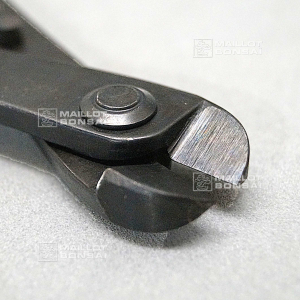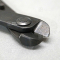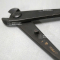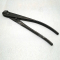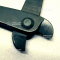ref. : 10749

45,00

Available quantity : 4Order

###### Description

Pincers for aluminium and copper thread. Japanese manufacture. Length: 205 millimetres. Thanks to the cutter’s shape they allow easy access the difficult places, with a perpendicular cut to the blades. Ergonomic handles. These cutters are intended for cutting 3mm thread or wire. They should not be used to cut barbed wire.

#wire 4.4 #cutters 3.7 #cutter 3.6 #thread 2.6 #they 2.4 #perpendicular 2.3 #millimetres 2.1 #manufacture 2.1 #ergonomic 1.9 #difficult 1.9

Formule
(( ROUND((CHAR_LENGTH(b.article_nom)-CHAR_LENGTH(REPLACE(b.article_nom, 'wire', '')))/LENGTH('wire')) + ROUND((CHAR_LENGTH(b.article_description)-CHAR_LENGTH(REPLACE(b.article_description, 'wire', '')))/LENGTH('wire')) ) * 4.4) + (( ROUND((CHAR_LENGTH(b.article_nom)-CHAR_LENGTH(REPLACE(b.article_nom, 'cutter', '')))/LENGTH('cutter')) + ROUND((CHAR_LENGTH(b.article_description)-CHAR_LENGTH(REPLACE(b.article_description, 'cutter', '')))/LENGTH('cutter')) ) * 3.6) + (( ROUND((CHAR_LENGTH(b.article_nom)-CHAR_LENGTH(REPLACE(b.article_nom, 'thread', '')))/LENGTH('thread')) + ROUND((CHAR_LENGTH(b.article_description)-CHAR_LENGTH(REPLACE(b.article_description, 'thread', '')))/LENGTH('thread')) ) * 2.6) + (( ROUND((CHAR_LENGTH(b.article_nom)-CHAR_LENGTH(REPLACE(b.article_nom, 'they', '')))/LENGTH('they')) + ROUND((CHAR_LENGTH(b.article_description)-CHAR_LENGTH(REPLACE(b.article_description, 'they', '')))/LENGTH('they')) ) * 2.4) + (( ROUND((CHAR_LENGTH(b.article_nom)-CHAR_LENGTH(REPLACE(b.article_nom, 'perpendicular', '')))/LENGTH('perpendicular')) + ROUND((CHAR_LENGTH(b.article_description)-CHAR_LENGTH(REPLACE(b.article_description, 'perpendicular', '')))/LENGTH('perpendicular')) ) * 2.3) + (( ROUND((CHAR_LENGTH(b.article_nom)-CHAR_LENGTH(REPLACE(b.article_nom, 'millimetres', '')))/LENGTH('millimetres')) + ROUND((CHAR_LENGTH(b.article_description)-CHAR_LENGTH(REPLACE(b.article_description, 'millimetres', '')))/LENGTH('millimetres')) ) * 2.1) + (( ROUND((CHAR_LENGTH(b.article_nom)-CHAR_LENGTH(REPLACE(b.article_nom, 'manufacture', '')))/LENGTH('manufacture')) + ROUND((CHAR_LENGTH(b.article_description)-CHAR_LENGTH(REPLACE(b.article_description, 'manufacture', '')))/LENGTH('manufacture')) ) * 2.1) + (( ROUND((CHAR_LENGTH(b.article_nom)-CHAR_LENGTH(REPLACE(b.article_nom, 'ergonomic', '')))/LENGTH('ergonomic')) + ROUND((CHAR_LENGTH(b.article_description)-CHAR_LENGTH(REPLACE(b.article_description, 'ergonomic', '')))/LENGTH('ergonomic')) ) * 1.9) + (( ROUND((CHAR_LENGTH(b.article_nom)-CHAR_LENGTH(REPLACE(b.article_nom, 'difficult', '')))/LENGTH('difficult')) + ROUND((CHAR_LENGTH(b.article_description)-CHAR_LENGTH(REPLACE(b.article_description, 'difficult', '')))/LENGTH('difficult')) ) * 1.9) + (( ROUND((CHAR_LENGTH(b.article_nom)-CHAR_LENGTH(REPLACE(b.article_nom, 'aluminium', '')))/LENGTH('aluminium')) + ROUND((CHAR_LENGTH(b.article_description)-CHAR_LENGTH(REPLACE(b.article_description, 'aluminium', '')))/LENGTH('aluminium')) ) * 1.9)

## Secure payment## Delivery

Our logistic partners :04 74 55 23 48
Pépinière MAILLOT-BONSAÏ
Le Bois Frazy
01990 RELEVANT - FRANCE
on appointment